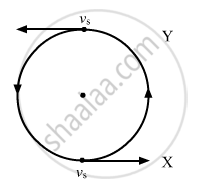Department of Pre-University Education, KarnatakaPUC Karnataka Science Class 11
Advertisement Remove all ads

# A Small Source of Sound Vibrating at Frequency 500 Hz is Rotated in a Circle of Radius 100/π Cm at a Constant Angular Speed of 5.0 Revolutions per Second - Physics

Sum

A small source of sound vibrating at frequency 500 Hz is rotated in a circle of radius 100/π cm at a constant angular speed of 5.0 revolutions per second. A listener situation situates himself in the plane of the circle. Find the minimum and the maximum frequency of the sound observed. Speed of sound in air = 332 m s−1.

Advertisement Remove all ads

#### Solution

Given:
Speed of sound in air v = 332 ms−1
Radius of the circle r = $\frac{100}{\pi}$ cm =$\frac{1}{\pi}$  m
Frequency of sound of the source $f_0$= 500 Hz
Angular speed $\omega$= 5 rev/s
Linear speed of the source is given by:

$v = \omega r$

⇒ $v = 5 \times \frac{1}{\pi} = \frac{5}{\pi} = 1 . 59 \text { m/s }$

∴ velocity of source $v_s$= 1.59 m/s
Let X be the position where the observer will listen at a maximum and Y be the position where he will listen at the minimum frequency.Apparent frequency $\left( f_1 \right)$at X is given by :

$f_1 = \left( \frac{v}{v - v_s} \right) f_0$

On substituting the values in the above equation, we get:

$f_1 = \left( \frac{332}{332 - 1 . 59} \right) \times 500 \approx 515 \text { Hz }$

Apparent frequency $\left( f_2 \right)$ at Y is given by:

$f_2 = \left( \frac{v}{v + v_s} \right) f_0$

On substituting the values in the above equation, we get:

$f_2 = \left( \frac{332}{332 + 1 . 59} \right) \times 500 \approx 485 \text{ Hz }$
Concept: Speed of Wave Motion
Is there an error in this question or solution?
Advertisement Remove all ads

#### APPEARS IN

HC Verma Class 11, 12 Concepts of Physics 1
Chapter 16 Sound Waves
Q 72 | Page 356
Advertisement Remove all ads
Advertisement Remove all ads
Share
Notifications

View all notifications

Forgot password?